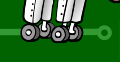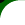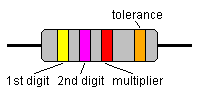HomeAboutKitsPICsDownloadsProblemsRough GuideClubMadDiaryMadLab AustraliaMadLab CanadaLinksContactLESSON 2  -  RESISTORS

Electrons move more easily through some materials than others when a voltage is applied. In metals the electrons are held so loosely that they move almost without any hindrance. We measure how much opposition there is to an electric current as resistance.

Resistors come somewhere between conductors, which conduct easily, and insulators, which don't conduct at all. Resistance is measured in ohms after the discoverer of a law relating voltage to current. Ohms are represented by the Greek letter omega.

Think back to the model of water flowing in a pipe. The thickness of the pipe must represent the resistance. The narrower the pipe the harder it is for the water to get through and hence the greater the resistance. For a particular pump the time taken to fill the pond is directly related to the pipe thickness. Make the pipe twice the size and the flow rate doubles, and the pond fills in half the time.

The resistors used in the MadLab kits are made of a thin film of carbon deposited on a ceramic rod. The less carbon the higher the resistance. They are then given a tough outer coating and some coloured bands are painted on.

The main function of resistors in a circuit is to control the flow of current to other components. Take an LED (light) for example. If too much current flows through an LED it is destroyed. So a resistor is used to limit the current.

When a current flows through a resistor energy is wasted and the resistor heats up. The greater the resistance the hotter it gets. The battery has to do work to force the electrons through the resistor and this work ends up as heat energy in the resistor.

An important property to know about a resistor is how much heat energy it can withstand before it's damaged. MadLab resistors can dissipate about a 1/4 Watt of heat (compare this with a domestic kettle which uses up to 3 000 Watts to boil water).

It's difficult to make a resistor to an exact value (and in most circuits it is not critical anyway). Resistances are given with a certain accuracy or tolerance. This is expressed as being plus or minus so much of a percentage. A 10% resistor with a stated value of 100 ohms could have a resistance anywhere between 90 ohms and 110 ohms. The MadLab resistors are 5% (that's what the gold band means) which is more than enough accuracy.

Real resistances vary over an enormous range. In the Lie Detector there is a 1 000 000 ohms resistor alongside a 470 ohms resistor. In circuit diagrams you will often see an 'R' instead of omega to represent ohms. This is a convention that dates from before the days of computers and laser printers when Greek letters were rarely found on typewriters. The letter 'k' means a thousand and its position shows the position of the decimal point.

Here are some examples:

```     10R = 10 ohms
10k = 10 kilohms = 10 000 ohms
4k7 = 4.7 kilohms = 4 700 ohms
```

Ohm's Law

Ohm's Law is actually very simple. It says that the more voltage applied to a resistor the more current flows through it. If the voltage is doubled then the current doubles, if the voltage is trebled then the current trebles, and so on. There is always a constant ratio between the voltage and current for a particular resistor. This value is the resistance measured in ohms.

To work out the resistance of something, simply measure the voltage across it and the current through it. Divide the first figure by the second and you have the resistance.

If you know the resistance and the voltage you can work out the current. Or if you know the resistance and the current you can work out the voltage. That makes Ohm's Law very useful.

Resistor Colour Code

The resistor colour code is a way of showing the value of a resistor. Instead of writing the resistance on its body, which would often be too small to read, a colour code is used. Ten different colours represent the numbers 0 to 9. The first two coloured bands on the body are the first two digits of the resistance, and the third band is the 'multiplier'. Multiplier just means the number of zeroes to add after the first two digits. Red represents the number 2, so a resistor with red, red, red bands has a resistance of 2 followed by 2 followed by 2 zeroes, which is 2 200 ohms or 2.2 kilohms.

The final band is the tolerance (the accuracy). All the MadLab resistors are 5% which is shown by a gold band.Here is the complete list of colours:

```                1st band     2nd band     3rd band
Black      0            0            x 1
Brown      1            1            x 10
Red        2            2            x 100
Orange     3            3            x 1000
Yellow     4            4            x 10000
Green      5            5            x 100000
Blue       6            6            x 1000000
Purple     7            7
Grey       8            8
White      9            9
```

Here are some examples:

```     Yellow, purple, red, gold = 47 x 100 = 4 700 ohms = 4.7 kilohms
Brown, black, yellow, gold = 10 x 10 000 = 100 kilohms
Yellow, purple, black, gold = 47 x 1 = 47 ohms
Brown, black, red, gold = 10 x 100 = 1 000 ohms = 1 kilohms
Brown, black, green, gold = 10 x 100 000 = 1 000 kilohms = 1 Megohms
All +/- 5%
```

Variable Resistors

Unsurprisingly, variable resistors are resistors whose resistance can be varied. The MadLab variable resistors (called presets) have a metal wiper resting on a circular track of carbon. The wiper moves along the track as the preset is turned. The current flows through the wiper and then through part of the carbon track. The more of the track it has to go through the greater the resistance.

MadLab presets have three legs. The top leg connects to the wiper and the other two legs to the two ends of the track. Generally only one of the track legs is actually used.

Variable resistors are used in circuits to vary things that need changing, like volume etc.

NEXT LESSON | CONTENTSHome | Documents | Source | Schematics | Contact | Order | Credits | Sitemap top of page